# simulate

Simulate posterior draws of Bayesian state-space model parameters

## Syntax

[Params,accept] = simulate(PriorMdl,Y,params0,Proposal)
[Params,accept] = simulate(PriorMdl,Y,params0,Proposal,Name=Value)

## Description

example

[Params,accept] = simulate(PriorMdl,Y,params0,Proposal) returns 1000 random vectors of state-space model parameters Params drawn from the posterior distribution Π(θ|Y), where PriorMdl specifies the prior distribution and data likelihood, and Y is the observed response data. params0 is the set of initial parameter values and Proposal is the covariance matrix of the proposal distribution of the Metropolis-Hastings sampler . accept is the acceptance rate of the proposal draws.

example

[Params,accept] = simulate(PriorMdl,Y,params0,Proposal,Name=Value) specifies options using one or more name-value arguments. For example, simulate(PriorMdl,Y,params0,Proposal,NumDraws=1e6,Thin=3,Dof=10) uses the multivariate t10 distribution for the Metropolis-Hastings proposal, draws 3e6 random vectors of parameters, and thins the sample to reduce serial correlation by discarding every 2 draws until it retains 1e6 draws.

## Examples

collapse all

Simulate observed responses from a known state-space model, then treat the model as Bayesian and draw parameters from the posterior distribution.

Suppose the following state-space model is a data-generating process (DGP).

$\left[\begin{array}{c}{x}_{t,1}\\ {x}_{t,2}\end{array}\right]=\left[\begin{array}{cc}0.5& 0\\ 0& -0.75\end{array}\right]\left[\begin{array}{c}{x}_{t-1,1}\\ {x}_{t-1,2}\end{array}\right]+\left[\begin{array}{cc}1& 0\\ 0& 0.5\end{array}\right]\left[\begin{array}{c}{u}_{t,1}\\ {u}_{t,2}\end{array}\right]$

${y}_{t}=\left[\begin{array}{cc}1& 1\end{array}\right]\left[\begin{array}{c}{x}_{t,1}\\ {x}_{t,2}\end{array}\right].$

Create a standard state-space model object ssm that represents the DGP.

trueTheta = [0.5; -0.75; 1; 0.5]; A = [trueTheta(1) 0; 0 trueTheta(2)]; B = [trueTheta(3) 0; 0 trueTheta(4)]; C = [1 1]; DGP = ssm(A,B,C);

Simulate a response path from the DGP.

rng(1); % For reproducibility y = simulate(DGP,200);

Suppose the structure of the DGP is known, but the state parameters trueTheta are unknown, explicitly

$\left[\begin{array}{c}{x}_{t,1}\\ {x}_{t,2}\end{array}\right]=\left[\begin{array}{cc}{\varphi }_{1}& 0\\ 0& {\varphi }_{2}\end{array}\right]\left[\begin{array}{c}{x}_{t-1,1}\\ {x}_{t-1,2}\end{array}\right]+\left[\begin{array}{cc}{\sigma }_{1}& 0\\ 0& {\sigma }_{2}\end{array}\right]\left[\begin{array}{c}{u}_{t,1}\\ {u}_{t,2}\end{array}\right]$

${y}_{t}=\left[\begin{array}{cc}1& 1\end{array}\right]\left[\begin{array}{c}{x}_{t,1}\\ {x}_{t,2}\end{array}\right].$

Consider a Bayesian state-space model representing the model with unknown parameters. Arbitrarily assume that the prior distribution of ${\varphi }_{1}$, ${\varphi }_{2}$, ${\sigma }_{1}^{2}$, and ${\sigma }_{2}^{2}$ are independent Gaussian random variables with mean 0.5 and variance 1.

The Local Functions section contains two functions required to specify the Bayesian state-space model. You can use the functions only within this script.

The paramMap function accepts a vector of the unknown state-space model parameters and returns all the following quantities:

• A = $\left[\begin{array}{cc}{\varphi }_{1}& 0\\ 0& {\varphi }_{2}\end{array}\right]$.

• B = $\left[\begin{array}{cc}{\sigma }_{1}& 0\\ 0& {\sigma }_{2}\end{array}\right]$.

• C = $\left[\begin{array}{cc}1& 1\end{array}\right]$.

• D = 0.

• Mean0 and Cov0 are empty arrays [], which specify the defaults.

• StateType = $\left[\begin{array}{cc}0& 0\end{array}\right]$, indicating that each state is stationary.

The paramDistribution function accepts the same vector of unknown parameters as does paramMap, but it returns the log prior density of the parameters at their current values. Specify that parameter values outside the parameter space have log prior density of -Inf.

Create the Bayesian state-space model by passing function handles directly to paramMap and paramDistribution to bssm.

Mdl = bssm(@paramMap,@priorDistribution)
Mdl = Mapping that defines a state-space model: @paramMap Log density of the prior distribution: @priorDistribution 

The simulate function requires a proposal distribution scale matrix. You can obtain a data-driven proposal scale matrix by using the tune function. Alternatively, you can supply your own scale matrix.

Obtain a data-driven scale matrix by using the tune function. Supply a random set of initial parameter values, and shut off the estimation summary display.

numParams = 4; theta0 = rand(numParams,1); [theta0,Proposal] = tune(Mdl,y,theta0,Display=false);
Local minimum found. Optimization completed because the size of the gradient is less than the value of the optimality tolerance. 

Draw 1000 random parameter vectors from the posterior distribution. Specify the simulated response path as observed responses and the optimized values returned by tune for the initial parameter values and the proposal distribution.

[Theta,accept] = simulate(Mdl,y,theta0,Proposal); accept
accept = 0.4010 

Theta is a 4-by-1000 matrix of randomly drawn parameters from the posterior distribution. Rows correspond to the elements of the input argument theta of the functions paramMap and priorDistribution.

accept is the proposal acceptance probability. In this case, simulate accepts 40% of the proposal draws.

Create trace plots of the parameters.

paramNames = ["\phi_1" "\phi_2" "\sigma_1" "\sigma_2"]; figure h = tiledlayout(4,1); for j = 1:numParams nexttile plot(Theta(j,:)) hold on yline(trueTheta(j)) ylabel(paramNames(j)) end title(h,"Posterior Trace Plots")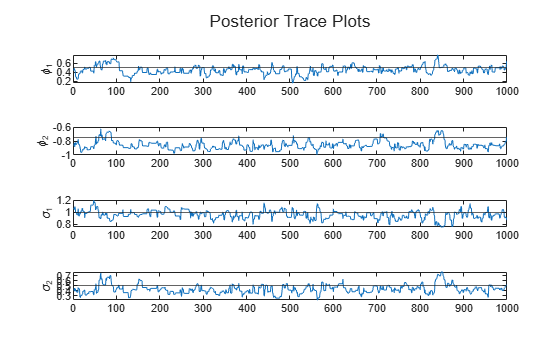The sampler eventually settles at near the true values of the parameters. In this case, the sample shows serial correlation and transient behavior. You can remedy serial correlation in the sample by adjusting the Thin name-value argument, and you can remedy transient effects by increasing the burn-in period using the BurnIn name-value argument.

Local Functions

This example uses the following functions. paramMap is the parameter-to-matrix mapping function and priorDistribution is the log prior distribution of the parameters.

function [A,B,C,D,Mean0,Cov0,StateType] = paramMap(theta) A = [theta(1) 0; 0 theta(2)]; B = [theta(3) 0; 0 theta(4)]; C = [1 1]; D = 0; Mean0 = []; % MATLAB uses default initial state mean Cov0 = []; % MATLAB uses initial state covariances StateType = [0; 0]; % Two stationary states end function logprior = priorDistribution(theta) paramconstraints = [(abs(theta(1)) >= 1) (abs(theta(2)) >= 1) ... (theta(3) < 0) (theta(4) < 0)]; if(sum(paramconstraints)) logprior = -Inf; else mu0 = 0.5*ones(numel(theta),1); sigma0 = 1; p = normpdf(theta,mu0,sigma0); logprior = sum(log(p)); end end

Consider the model in the example Draw Random Parameters from Posterior Distribution of Time-Invariant Model. Improve the Markov chain convergence by adjusting sampler options.

Create a standard state-space model object ssm that represents the DGP, and then simulate a response path.

trueTheta = [0.5; -0.75; 1; 0.5]; A = [trueTheta(1) 0; 0 trueTheta(2)]; B = [trueTheta(3) 0; 0 trueTheta(4)]; C = [1 1]; DGP = ssm(A,B,C); rng(1); % For reproducibility y = simulate(DGP,200);

Create the Bayesian state-space model by passing function handles directly to paramMap and paramDistribution to bssm (the functions are in Local Functions).

Mdl = bssm(@paramMap,@priorDistribution)
Mdl = Mapping that defines a state-space model: @paramMap Log density of the prior distribution: @priorDistribution 

Simulate random parameter vectors from the posterior distribution. Specify the simulated response path as observed responses, and obtain an optimal proposal distribution by using tune and shut off all optimization displays. The plots in Draw Random Parameters from Posterior Distribution of Time-Invariant Model suggest that the Markov chain settles after 500 draws. Therefore, specify a burn-in period of 500 (BurnIn=500). Specify thinning the sample by keeping the first draw of each set of 30 successive draws (Thin=30). Retain 2000 random parameter vectors (NumDraws=2000).

numParams = 4; theta0 = rand(numParams,1); options = optimoptions("fminunc",Display="off"); [theta0,Proposal] = tune(Mdl,y,theta0,Display=false,Options=options); [Theta,accept] = simulate(Mdl,y,theta0,Proposal, ... NumDraws=2000,BurnIn=500,Thin=30); accept
accept = 0.3885 

Theta is a 4-by-2000 matrix of randomly drawn parameters from the posterior distribution. Rows correspond to the elements of the input argument theta of the functions paramMap and priorDistribution.

accept is the proposal acceptance probability. In this case, simulate accepts 39% of the proposal draws.

Create trace plots and correlograms of the parameters.

paramNames = ["\phi_1" "\phi_2" "\sigma_1" "\sigma_2"]; figure h = tiledlayout(4,1); for j = 1:numParams nexttile plot(Theta(j,:)) hold on yline(trueTheta(j)) ylabel(paramNames(j)) end title(h,"Posterior Trace Plots")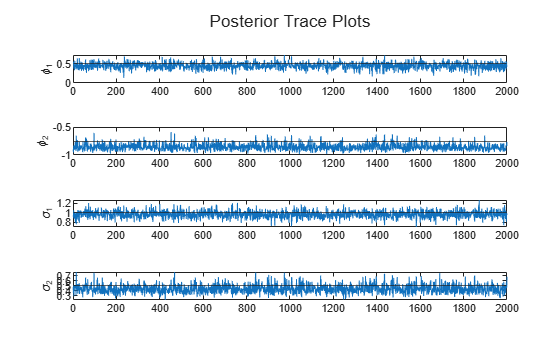figure h = tiledlayout(4,1); for j = 1:numParams nexttile autocorr(Theta(j,:)); ylabel(paramNames(j)); title([]); end title(h,"Posterior Sample Correlograms")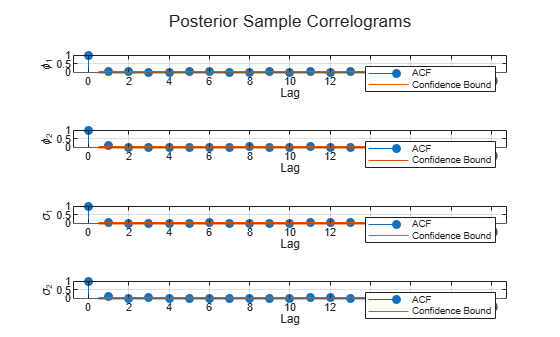The sampler quickly settles near the true values of the parameters. The sample shows little serial correlation and no transient behavior.

Local Functions

This example uses the following functions. paramMap is the parameter-to-matrix mapping function and priorDistribution is the log prior distribution of the parameters.

function [A,B,C,D,Mean0,Cov0,StateType] = paramMap(theta) A = [theta(1) 0; 0 theta(2)]; B = [theta(3) 0; 0 theta(4)]; C = [1 1]; D = 0; Mean0 = []; % MATLAB uses default initial state mean Cov0 = []; % MATLAB uses initial state covariances StateType = [0; 0]; % Two stationary states end function logprior = priorDistribution(theta) paramconstraints = [(abs(theta(1)) >= 1) (abs(theta(2)) >= 1) ... (theta(3) < 0) (theta(4) < 0)]; if(sum(paramconstraints)) logprior = -Inf; else mu0 = 0.5*ones(numel(theta),1); sigma0 = 1; p = normpdf(theta,mu0,sigma0); logprior = sum(log(p)); end end

Consider the following time-varying, state-space model for a DGP:

• From periods 1 through 250, the state equation includes stationary AR(2) and MA(1) models, respectively, and the observation model is the weighted sum of the two states.

• From periods 251 through 500, the state model includes only the first AR(2) model.

• ${\mu }_{0}=\left[\begin{array}{cccc}0.5& 0.5& 0& 0\end{array}\right]$ and ${\Sigma }_{0}$ is the identity matrix.

Symbolically, the DGP is

$\begin{array}{l}\begin{array}{c}\left[\begin{array}{c}{x}_{1t}\\ {x}_{2t}\\ {x}_{3t}\\ {x}_{4t}\end{array}\right]=\left[\begin{array}{cccc}{\varphi }_{1}& {\varphi }_{2}& 0& 0\\ 1& 0& 0& 0\\ 0& 0& 0& \theta \\ 0& 0& 0& 0\end{array}\right]\left[\begin{array}{c}{x}_{1,t-1}\\ {x}_{2,t-1}\\ {x}_{3,t-1}\\ {x}_{4,t-1}\end{array}\right]+\left[\begin{array}{cc}{\sigma }_{1}& 0\\ 0& 0\\ 0& 1\\ 0& 1\end{array}\right]\left[\begin{array}{c}{u}_{1t}\\ {u}_{2t}\end{array}\right]\\ {y}_{t}={c}_{1}\left({x}_{1t}+{x}_{3t}\right)+{\sigma }_{2}{\epsilon }_{t}.\end{array}\phantom{\rule{0.2777777777777778em}{0ex}}for\phantom{\rule{0.2777777777777778em}{0ex}}t=1,...,250,\\ \begin{array}{c}\left[\begin{array}{c}{x}_{1t}\\ {x}_{2t}\end{array}\right]=\left[\begin{array}{cccc}{\varphi }_{1}& {\varphi }_{2}& 0& 0\\ 1& 0& 0& 0\end{array}\right]\left[\begin{array}{c}{x}_{1,t-1}\\ {x}_{2,t-1}\\ {x}_{3,t-1}\\ {x}_{4,t-1}\end{array}\right]+\left[\begin{array}{c}{\sigma }_{1}\\ 0\end{array}\right]{u}_{1t}\\ {y}_{t}={c}_{2}{x}_{1t}+{\sigma }_{3}{\epsilon }_{t}.\end{array}\phantom{\rule{0.2777777777777778em}{0ex}}for\phantom{\rule{0.2777777777777778em}{0ex}}t=251,\\ \begin{array}{c}\left[\begin{array}{c}{x}_{1t}\\ {x}_{2t}\end{array}\right]=\left[\begin{array}{cc}{\varphi }_{1}& {\varphi }_{2}\\ 1& 0\end{array}\right]\left[\begin{array}{c}{x}_{1,t-1}\\ {x}_{2,t-1}\end{array}\right]+\left[\begin{array}{c}{\sigma }_{1}\\ 0\end{array}\right]{u}_{1t}\\ {y}_{t}={c}_{2}{x}_{1t}+{\sigma }_{3}{\epsilon }_{t}.\end{array}\phantom{\rule{0.2777777777777778em}{0ex}}for\phantom{\rule{0.2777777777777778em}{0ex}}t=252,...,500.\end{array}$

where:

• The AR(2) parameters $\left\{{\varphi }_{1},{\varphi }_{2}\right\}=\left\{0.5,-0.2\right\}$ and ${\sigma }_{1}=0.4$.

• The MA(1) parameter $\theta =0.3$.

• The observation equation parameters $\left\{{\mathit{c}}_{1},{\mathit{c}}_{2}\right\}=\left\{2,3\right\}$ and $\left\{{\sigma }_{2},{\sigma }_{3}\right\}=\left\{0.1,0.2\right\}$.

Simulate a response path of length 500 from the model. The function timeVariantParamMapBayes.m, stored in matlabroot/examples/econ/main, specifies the state-space model structure.

params = [0.5; -0.2; 0.4; 0.3; 2; 0.1; 3; 0.2]; numObs = 500; numParams = numel(params); [A,B,C,D,mean0,Cov0,stateType] = timeVariantParamMapBayes(params,numObs); DGP = ssm(A,B,C,D,Mean0=mean0,Cov0=Cov0,StateType=stateType); rng(1) % For reproducibility y = simulate(DGP,numObs); plot(y) ylabel("y")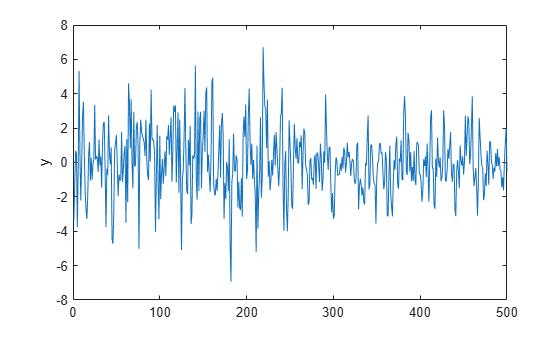Create a time-varying, Bayesian state-space model that uses the structure of the DGP, but all parameters are unknown and the prior density is flat (the function flatPriorBSSM.m in matlabroot/examples/econ/main is the prior density).

Create a bssm object representing the Bayesian state-space model object.

Mdl = bssm(@(params)timeVariantParamMapBayes(params,numObs),@flatPriorBSSM);

Draw a sample from the posterior distribution. Initialize the parameter values to a random set of positive values in [0,0.5]. Set the proposal distribution to multivariate ${\mathit{t}}_{25}$ with a scale matrix proportional. Set the proportionality constant to 0.005.

params0 = 0.5*rand(numParams,1); options = optimoptions("fminunc",Display="off"); [params0,Proposal] = tune(Mdl,y,params0,Options=options,Display=false); [PostParams,accept] = simulate(Mdl,y,params0,Proposal, ... Dof=25,Proportion=0.005); accept
accept = 0.7460 

PostParams is an 8-by-1000 matrix of 1000 random draws from the posterior distribution. The Metropolis-Hastings sampler accepted 75% of the proposed draws.

When you work with a state-space model that contains a deflated response variable, you must have data for the predictors.

Consider a regression of the US unemployment rate onto and real gross national product (rGNP) rate, and suppose the resulting innovations are an ARMA(1,1) process. The state-space form of the relationship is

$\begin{array}{l}\left[\begin{array}{c}{x}_{1,t}\\ {x}_{2,t}\end{array}\right]=\left[\begin{array}{cc}\varphi & \theta \\ 0& 0\end{array}\right]\left[\begin{array}{c}{x}_{1,t-1}\\ {x}_{2,t-1}\end{array}\right]+\left[\begin{array}{c}\sigma \\ 1\end{array}\right]{u}_{t}\\ {y}_{t}-\beta {Z}_{t}={x}_{1,t},\end{array}$

where:

• ${x}_{1,t}$ is the ARMA process.

• ${x}_{2,t}$ is a dummy state for the MA(1) effect.

• ${y}_{t}$ is the observed unemployment rate deflated by a constant and the rGNP rate (${Z}_{t}$).

• ${u}_{t}$ is an iid Gaussian series with mean 0 and standard deviation 1.

Load the Nelson-Plosser data set, which contains a table DataTable that has the unemployment rate and rGNP series, among other series.

load Data_NelsonPlosser

Create a variable in DataTable that represents the returns of the raw rGNP series. Because price-to-returns conversion reduces the sample size by one, prepad the series with NaN.

DataTable.RGNPRate = [NaN; price2ret(DataTable.GNPR)]; T = height(DataTable);

Create variables for the regression. Represent the unemployment rate as the observation series and the constant and rGNP rate series as the deflation data ${\mathit{Z}}_{\mathit{t}}$.

Z = [ones(T,1) DataTable.RGNPRate]; y = DataTable.UR;

The functions armaDeflateYBayes.m and flatPriorDeflateY.m in matlabroot/examples/econ/main specify the state-space model structure and likelihood, and the flat prior distribution.

Create a bssm object representing the Bayesian state-space model. Specify the parameter-to-matrix mapping function as a handle to a function solely of the parameters.

numParams = 5; Mdl = bssm(@(params)armaDeflateYBayes(params,y,Z),@flatPriorDeflateY)
Mdl = Mapping that defines a state-space model: @(params)armaDeflateYBayes(params,y,Z) Log density of the prior distribution: @flatPriorDeflateY 

Draw a sample from the posterior distribution. Initialize the Kalman filter with a random set of positive values in [0,0.5]. Obtain an optimal set of proposal distribution moments for the sampler by using tune. Set the proportionality constant to 0.01. Set a burn-in period of 2000 draws, set a thinning factor of 50, and specify retaining 1000 draws.

rng(1) % For reproducibility params0 = 0.5*rand(numParams,1); options = optimoptions("fminunc",Display="off"); [params0,Proposal] = tune(Mdl,y,params0,Options=options, ... Display=false); [PostParams,accept] = simulate(Mdl,y,params0,Proposal,Proportion=0.01, ... BurnIn=2000,NumDraws=1000,Thin=50); accept
accept = 0.9200 

PostParams is a 5-by-1000 matrix of 1000 draws from the posterior distribution. The Metropolis-Hastings sampler accepts 92% of the proposed draws.

paramNames = ["\phi" "\theta" "\sigma" "\beta_0" "\beta_1"]; figure h = tiledlayout(numParams,1); for j = 1:numParams nexttile plot(PostParams(j,:)) hold on ylabel(paramNames(j)) end title(h,"Posterior Trace Plots")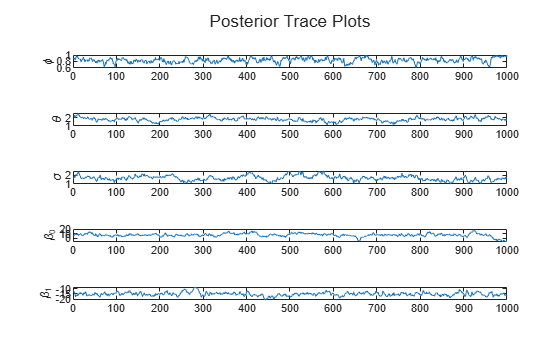All samples appear to suffer from autocorrelation. To improve the Markov chain, experiment with the Thin option.

## Input Arguments

collapse all

Prior Bayesian state-space model, specified as a bssm model object return by bssm or ssm2bssm.

The function handles of the properties PriorMdl.ParamDistribution and PriorMdl.ParamMap determine the prior and the data likelihood, respectively.

Observed response data, from which simulate forms the posterior distribution, specified as a numeric matrix or a cell vector of numeric vectors.

• If PriorMdl is time invariant with respect to the observation equation, Y is a T-by-n matrix. Each row of the matrix corresponds to a period and each column corresponds to a particular observation in the model. T is the sample size and n is the number of observations per period. The last row of Y contains the latest observations.

• If PriorMdl is time varying with respect to the observation equation, Y is a T-by-1 cell vector. Y{t} contains an nt-dimensional vector of observations for period t, where t = 1, ..., T. The corresponding dimensions of the coefficient matrices, outputs of PriorMdl.ParamMap, C{t}, and D{t} must be consistent with the matrix in Y{t} for all periods. The last cell of Y contains the latest observations.

NaN elements indicate missing observations. For details on how the Kalman filter accommodates missing observations, see Algorithms.

Data Types: double | cell

Initial parameter values, specified as a numParams-by-1 numeric vector. Elements of params0 must correspond to the elements of the first input arguments of PriorMdl.ParamMap and Mdl.ParamDistribution.

Data Types: double

Proposal distribution covariance/scale matrix for the Metropolis-Hastings sampler, up to the proportionality constant Proportion, specified as a numParams-by-numParams, positive-definite numeric matrix. Elements of Proposal must correspond to elements in params0.

The proposal distribution is multivariate normal or Student's t with Dof degrees of freedom (for details, see Dof).

Data Types: double

### Name-Value Arguments

Specify optional pairs of arguments as Name1=Value1,...,NameN=ValueN, where Name is the argument name and Value is the corresponding value. Name-value arguments must appear after other arguments, but the order of the pairs does not matter.

Before R2021a, use commas to separate each name and value, and enclose Name in quotes.

Example: simulate(Mdl,Y,params0,Proposal,NumDraws=1e6,Thin=3,Dof=10) uses the multivariate t10 distribution for the Metropolis-Hastings proposal, draws 3e6 random vectors of parameters, and thins the sample to reduce serial correlation by discarding every 2 draws until it retains 1e6 draws.

Number of Metropolis-Hastings sampler draws from the posterior distribution Π(θ|Y), specified as a positive integer.

Example: NumDraws=1e7

Data Types: double

Number of draws to remove from the beginning of the sample to reduce transient effects, specified as a nonnegative scalar. For details on how simulate reduces the full sample, see Algorithms.

Tip

1. Determine the extent of the transient behavior in the sample by setting the BurnIn name-value argument to 0.

2. Simulate a few thousand observations by using simulate.

3. Create trace plots.

Example: BurnIn=1000

Data Types: double

Adjusted sample size multiplier, specified as a positive integer.

The actual sample size is BurnIn + NumDraws*Thin. After discarding the burn-in, simulate discards every Thin1 draws, and then retains the next draw. For more details on how simulate reduces the full sample, see Algorithms.

Tip

To reduce potential large serial correlation in the posterior sample, or to reduce the memory consumption of the output sample, specify a large value for Thin.

Example: Thin=5

Data Types: double

Univariate treatment of a multivariate series flag, specified as a value in this table.

ValueDescription
trueApplies the univariate treatment of a multivariate series, also known as sequential filtering
falseDoes not apply sequential filtering

The univariate treatment can accelerate and improve numerical stability of the Kalman filter. However, all observation innovations must be uncorrelated. That is, DtDt' must be diagonal, where Dt (t = 1, ..., T) is the output coefficient matrix D of PriorMdl.ParamMap and PriorMdl.ParamDistribution.

Example: Univariate=true

Data Types: logical

Square root filter method flag, specified as a value in this table.

ValueDescription
trueApplies the square root filter method for the Kalman filter
falseDoes not apply the square root filter method

If you suspect that the eigenvalues of the filtered state or forecasted observation covariance matrices are close to zero, then specify SquareRoot=true. The square root filter is robust to numerical issues arising from the finite precision of calculations, but requires more computational resources.

Example: SquareRoot=true

Data Types: logical

Proposal distribution degrees of freedom for the Metropolis-Hastings sampler, specified as a value in this table.

ValueMetropolis-Hastings Proposal Distribution
Positive scalarMultivariate t with Dof degrees of freedom
InfMultivariate normal

The following options specify other aspects of the proposal distribution:

Example: Dof=10

Data Types: double

Proposal scale matrix proportionality constant, specified as a positive scalar.

Tip

For higher proposal acceptance rates, experiment with relatively small values for Proportion.

Example: Proportion=1

Data Types: double

Proposal distribution center, specified as a value in this table.

ValueDescription
numParams-by-1 numeric vectorsimulate uses the independence Metropolis-Hastings sampler. Center is the center of the proposal distribution.
[] (empty array)simulate uses the random-walk Metropolis-Hastings sampler. The center of the proposal density is the current state of the Markov chain.

Example: Center=ones(10,1)

Data Types: double

## Output Arguments

collapse all

Posterior draws of the state-space model parameters, returned as a numParams-by-NumDraws numeric matrix. Each column is a separate draw from the distribution. Each row corresponds to the corresponding element of params0.

Because the Metropolis-Hastings sampler is a Markov chain, successive draws are correlated.

Proposal acceptance rate, returned as a positive scalar in [0,1].

## Tips

• Acceptance rates from accept that are relatively close to 0 or 1 indicate that the Markov chain does not sufficiently explore the posterior distribution. To obtain an appropriate acceptance rate for your data, tune the sampler by implementing one of the following procedures.

• Use the tune function to search for the posterior mode by numerical optimization. tune returns optimized parameters and the proposal scale matrix, which is proportional to the negative Hessian matrix evaluated at the posterior mode. Pass the optimized parameters and the proposal scale to simulate, and tune the proportionality constant by using the Proportion name-value argument. Small values of Proportion tend to increase the proposal acceptance rates of the Metropolis-Hastings sampler.

• Perform a multistage simulation:

1. Choose an initial value for the input Proposal and simulate draws from the posterior.

2. Compute the sample covariance matrix, and pass the result to simulate as an updated value for Proposal.

3. Repeat steps 1 and 2 until accept is reasonable for your data.

## Algorithms

This figure shows how simulate reduces the sample by using the values of NumDraws, Thin, and BurnIn. Rectangles represent successive draws from the distribution. simulate removes the white rectangles from the sample. The remaining NumDraws black rectangles compose the sample.Hastings, Wilfred K. "Monte Carlo Sampling Methods Using Markov Chains and Their Applications." Biometrika 57 (April 1970): 97–109. https://doi.org/10.1093/biomet/57.1.97.

 Metropolis, Nicholas, Rosenbluth, Arianna. W., Rosenbluth, Marshall. N., Teller, Augusta. H., and Teller, Edward. "Equation of State Calculations by Fast Computing Machines." The Journal of Chemical Physics 21 (June 1953): 1087–92. https://doi.org/10.1063/1.1699114.